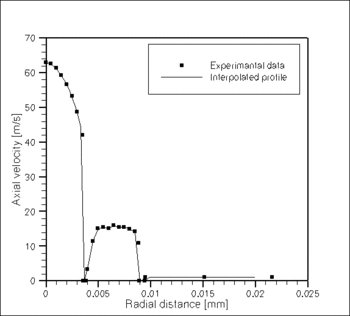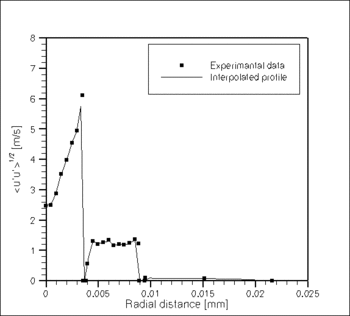# Overview of Tests

The velocity measurements were performed with two-component fiber-optic laser Doppler anemometer (Dantec). All the details of the flow field measuring techniques applied in Sandia Flame D experiment are explained in. Measured scalars for Sandia D Flame include temperature, mixture fraction, N2, O2, H2O, H2, CH4, CO, CO2, OH and NO. Experimental methods and measurement uncertainties are outlined in Spontaneous Raman scattering of the beams from two Nd:YAG lasers (532 nm) was used to measure concentrations of the major species. The Rayleigh scattering signal was converted to temperature using a species-weighted scattering cross section, based on the Raman measurements. Linear laser-induced fluorescence (LIF) was used to measure OH and NO, and the fluorescence signals were corrected on a shot-to-shot basis for variations in Boltzmann fraction and collisional quenching rate. The concentration of CO was measured by Raman scattering and by two-photon laser-induced fluorescence (TPLIF).

Table EXP – A  Summary description of all test cases
Name GNDPs PDPs (Problem Definition Parameters) MPs (Measured Parameters)
Re Fuel jet composition Pilot flame composition Detailed data DOAPs
EXP1 22400 25% of methane (CH4) and 75% of air C2H2, H2, air, CO2 and N2

$\langle U\rangle ,\langle U_{rms}\rangle ,\langle V\rangle ,\langle V_{rms}\rangle ,\langle \eta \rangle ,$$\langle T\rangle ,\langle Y_{H_{2}0}\rangle ,\langle Y_{O_{2}}\rangle ,\langle Y_{N_{2}}\rangle ,\langle Y_{H_{2}}\rangle ,$$\langle Y_{CO}\rangle ,\langle Y_{CO_{2}}\rangle ,\langle Y_{CH_{4}}\rangle ,\langle \eta _{rms}\rangle ,$$\langle T_{rms}\rangle ,\langle Y_{{H_{2}O}_{rms}}\rangle ,\langle Y_{{O_{2}}_{rms}}\rangle ,$$\langle Y_{{N_{2}}_{rms}}\rangle ,\langle Y_{{H_{2}}_{rms}}\rangle ,\langle Y_{{CO}_{rms}}\rangle ,$$\langle Y_{{CO_{2}}_{rms}}\rangle ,\langle Y_{{CH_{4}}_{rms}}\rangle$Axial profiles

Tmax , z/D (Tmax )

Lconst(η , YCH4 , YO2)

Lconst(YH2O , YCO2)

YH2, max , z/D (YH2, max )

YCO, max , z/D (YCO, max )

RMSmax

z/D (RMSmax )

x/D = 15, 30, 45

Fmax , Umax

r½(η) , r½(U )

Table EXP – B  Summary description of all measured parameters
MP1 MP2 MP3 DOAPs or other miscellaneous data
U, V, u ′, v ′ (ms-1) η, T, η ′, T ′ (m2s-2) YN2, RMS(YN2)

YO2, RMS(YO2)

YH2O, RMS(YH2O )

YH2, RMS(YH2)

YCH4, RMS(YCH4)

YCO, RMS(YCO )

YCO2, RMS(YCO2)

YOH, RMS(YOH )

YNO, RMS(YNO )

## TEST CASE EXP1

### Description of Experiment

The Application Challenge includes just one test case, Sandia Flame D with defined Reynolds number of the fuel jet and the fuel and pilot flame compositions as given in Table EXP-A.

### Boundary Data

The inlet mean and fluctuating velocity at the distance x/D=1 from the burner are shown in Fig.3. The inlet parabolic profile had a maximum at the centre of the fuel nozzle of Umax = 62 m/s. The pilot flame bulk velocity Upilot = 11.4 m/s and the coflow velocity Ucfl = 0.9 m/s.Fig. 3: Mean and RMS inlet profiles of the axial velocity.

### Measurement Errors

The flow field measurement statistical errors are estimated in as below 5% for the mean velocities and within 10% for fluctuating components. The scalar measurement errors are estimated and analyzed in. The relative uncertainty (not including statistical noise or potential effects of spatial averaging) is estimated to be within 2% for the Raman measurements, 5% for OH, 5% for CO, and 10% for NO.

### Measured Data

The velocity data (in ASCII format) can be obtained by contacting Prof. Andreas Dreizler, TU Darmstadt (dreizler@csi.tu-darmstadt.de).

The scalar data are available at http://www.sandia.gov/TNF/DataArch/FlameD.html